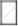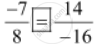Share

# Fill in the Boxes with the Correct Symbol Out of >, <, and = (-7)/8 and 4/(-16) - CBSE Class 7 - Mathematics

ConceptRational Numbers Between Two Rational Numbers

#### Question

Fill in the boxes with the correct symbol out of >, <, and =

(-7)/814/(-16)

#### Solution

Here  14/()-16 = (7xx2)/(-8xx2) = 7/(-8) = (-7)/8

ThereforeIs there an error in this question or solution?

#### APPEARS IN

NCERT Solution for Mathematics for Class 7 (2018 to Current)
Chapter 9: Rational Numbers
Ex. 9.10 | Q: 8.3 | Page no. 183
Solution Fill in the Boxes with the Correct Symbol Out of >, <, and = (-7)/8 and 4/(-16) Concept: Rational Numbers Between Two Rational Numbers.
S